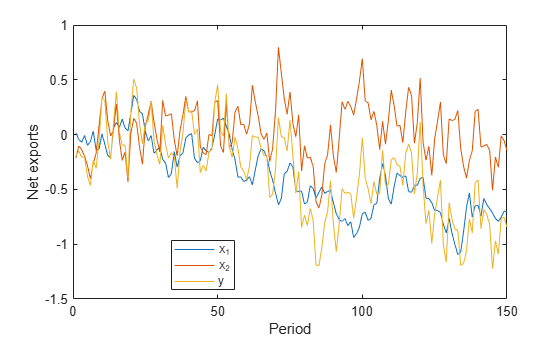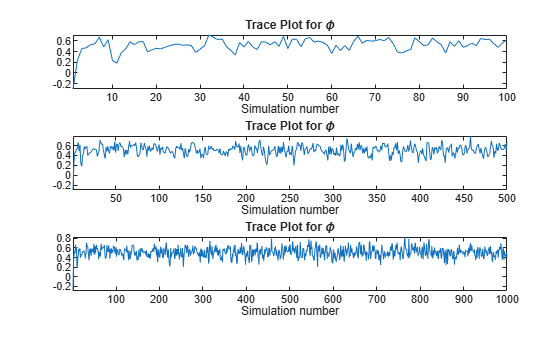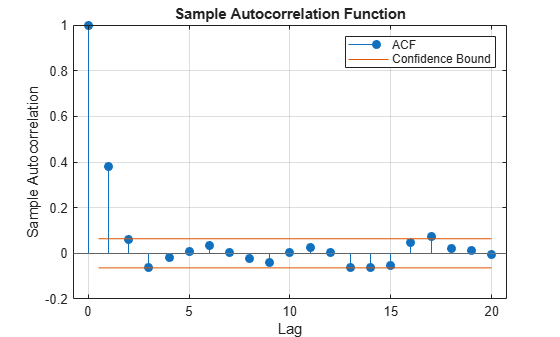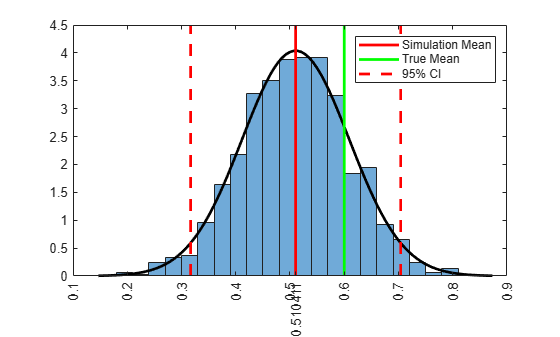# Estimate Random Parameter of State-Space Model

This example shows how to estimate a random, autoregressive coefficient of a state in a state-space model. That is, this example takes a Bayesian view of state-space model parameter estimation by using the "zig-zag" estimation method.

Suppose that two states (${x}_{1,t}$ and ${x}_{2,t}$) represent the net exports of two countries at the end of the year.

• ${x}_{1,t}$ is a unit root process with a disturbance variance of ${\sigma }_{1}^{2}$.

• ${x}_{2,t}$ is an AR(1) process with an unknown, random coefficient and a disturbance variance of ${\sigma }_{2}^{2}$.

• An observation (${y}_{t}$) is the exact sum of the two net exports. That is, the net exports of the individual states are unknown.

Symbolically, the true state-space model is

$\begin{array}{l}{x}_{1,t}={x}_{1,t-1}+{\sigma }_{1}{u}_{1,t}\\ {x}_{2,t}=\varphi {x}_{2,t-1}+{\sigma }_{2}{u}_{2,t}\\ {y}_{t}={x}_{1,t}+{x}_{2,t}\end{array}$

### Simulate Data

Simulate 100 years of net exports from:

• A unit root process with a mean zero, Gaussian noise series that has variance $0.{1}^{2}$.

• An AR(1) process with an autoregressive coefficient of 0.6 and a mean zero, Gaussian noise series that has variance $0.{2}^{2}$.

• ${x}_{1,0}={x}_{2,0}=0$.

• Create an observation series by summing the two net exports per year.

rng(100); % For reproducibility T = 150; sigma1 = 0.1; sigma2 = 0.2; phi = 0.6; u1 = randn(T,1)*sigma1; x1 = cumsum(u1); Mdl2 = arima('AR',phi,'Variance',sigma2^2,'Constant',0); x2 = simulate(Mdl2,T,'Y0',0); y = x1 + x2; figure; plot([x1 x2 y]) legend('x_1','x_2','y','Location','Best'); ylabel('Net exports'); xlabel('Period');### The Zig-Zag Estimation Method

Treat $\varphi$ as if it is unknown and random, and use the zig-zag method to recover its distribution. To implement the zig-zag method:

1. Choose an initial value for $\varphi$ in the interval (-1,1), and denote it ${\varphi }_{z}$.

2. Create the true state-space model, that is, an ssm model object that represents the data-generating process.

3. Use the simulation smoother (simsmooth) to draw a random path from the distribution of the second smoothed states. Symbolically, ${x}_{2,z,t}\sim P\left({x}_{2,t}|{y}_{t},{x}_{1,t},\varphi ={\varphi }_{z}\right)$.

4. Create another state-space model that has this form

$\begin{array}{l}{\varphi }_{z,t}={\varphi }_{z,t-1}\\ {x}_{2,z,t}={x}_{2,z,t-1}{\varphi }_{z,t}+0.8{u}_{2,t}\end{array}$

In words, ${\varphi }_{z,t}$ is a static state and ${x}_{2,z,t}$ is an "observed" series with time varying coefficient ${C}_{t}={x}_{2,z,t-1}$.

5. Use the simulation smoother to draw a random path from the distribution of the smoothed ${\varphi }_{z,t}$ series. Symbolically, ${\varphi }_{z,t}\sim P\left(\varphi |{x}_{2,z,t}\right)$, where ${x}_{2,z,t}$ encompasses the structure of the true state-space model and the observations. ${\varphi }_{z,t}$ is static, so you can just reserve one value (${\varphi }_{z}$).

6. Repeat steps 2 - 5 many times and store ${\varphi }_{z}$ each iteration.

7. Perform diagnostic checks on the simulation series. That is, construct:

• Trace plots to determine the burn in period and whether the Markov chain is mixing well.

• Autocorrelation plots to determine how many draws need removing to obtain a well-mixed Markov chain.

8. The remaining series represents draws from the posterior distribution of $\varphi$. You can compute descriptive statistics, or plot a histogram to determine the qualities of the distribution.

### Estimate Random Coefficient Using Zig-Zag Method

Specify initial values, preallocate, and create the true state-space model.

phi0 = -0.3; % Initial value of phi Z = 1000; % Number of times to iterate the zig-zag method phiz = [phi0; nan(Z,1)]; % Preallocate A = [1 0; 0 NaN]; B = [sigma1; sigma2]; C = [1 1]; Mdl = ssm(A,B,C,'StateType',[2; 0]);

Mdl is an ssm model object. The NaN acts as a placeholder for $\varphi$.

Iterate steps 2 - 5 of the zig-zag method.

for j = 2:(Z + 1); % Draw a random path from smoothed x_2 series. xz = simsmooth(Mdl,y,'Params',phiz(j-1)); % The second column of xz is a draw from the posterior distribution of x_2. % Create the intermediate state-space model. Az = 1; Bz = 0; Cz = num2cell(xz((1:(end - 1)),2)); Dz = sigma2; Mdlz = ssm(Az,Bz,Cz,Dz,'StateType',2); % Draw a random path from the smoothed phiz series. phizvec = simsmooth(Mdlz,xz(2:end,2)); phiz(j) = phizvec(1); % phiz(j) is a draw from the posterior distribution of phi end

phiz is a Markov chain. Before analyzing the posterior distribution of $\varphi$, you should assess whether to impose a burn-in period, or the severity of the autocorrelation in the chain.

### Determine Quality of Simulation

Draw a trace plot for the first 100, 500, and all of the random draws.

vec = [100 500 Z]; figure; for j = 1:3; subplot(3,1,j) plot(phiz(1:vec(j))); title('Trace Plot for \phi'); xlabel('Simulation number'); axis tight; endAccording to the first plot, transient effects die down after about 20 draws. Therefore, a short burn-in period should suffice. The plot of the entire simulation shows that the series settles around a center.

Plot the autocorrelation function of the series after removing the first 20 draws.

burnOut = 21:Z; figure; autocorr(phiz(burnOut));The autocorrelation function dies out rather quickly. It doesn't seem like autocorrelation in the chain is an issue.

Determine qualities of the posterior distribution of $\varphi$ by computing simulation statistics and by plotting a histogram of the reduced set of random draws.

xbar = mean(phiz(burnOut))
xbar = 0.5104 
xstd = std(phiz(burnOut))
xstd = 0.0988 
ci = norminv([0.025,0.975],xbar,xstd); % 95% confidence interval figure; histogram(phiz(burnOut),'Normalization','pdf'); h = gca; hold on; simX = linspace(h.XLim(1),h.XLim(2),100); simPDF = normpdf(simX,xbar,xstd); plot(simX,simPDF,'k','LineWidth',2); h1 = plot([xbar xbar],h.YLim,'r','LineWidth',2); h2 = plot([0.6 0.6],h.YLim,'g','LineWidth',2); h3 = plot([ci(1) ci(1)],h.YLim,'--r',... [ci(2) ci(2)],h.YLim,'--r','LineWidth',2); legend([h1 h2 h3(1)],{'Simulation Mean','True Mean','95% CI'}); h.XTick = sort([h.XTick xbar]); h.XTickLabel{h.XTick == xbar} = xbar; h.XTickLabelRotation = 90;The posterior distribution of $\varphi$ is roughly normal with mean and standard deviation approximately 0.51 and 0.1, respectively. The true mean of $\varphi$ is 0.6, and it is less than one standard deviation to the right of the simulation mean.

Compute the maximum likelihood estimate of $\varphi$. That is, treat $\varphi$ as a fixed, but unknown parameter, and then estimate Mdl using the Kalman filter and maximum likelihood.

[~,estParams] = estimate(Mdl,y,phi0)
Method: Maximum likelihood (fminunc) Sample size: 150 Logarithmic likelihood: -10.1434 Akaike info criterion: 22.2868 Bayesian info criterion: 25.2974 | Coeff Std Err t Stat Prob ------------------------------------------------------- c(1) | 0.53590 0.19183 2.79360 0.00521 | | Final State Std Dev t Stat Prob x(1) | -0.85059 0.00000 -6.45811e+08 0 x(2) | 0.00454 0 Inf 0 
estParams = 0.5359 

The MLE of $\varphi$ is 0.54. Both estimates are within one standard deviation or standard error from the true value of $\varphi$.